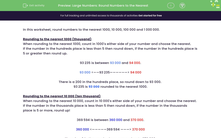# Practise Rounding Large Numbers

In this worksheet, students will round numbers to the nearest 1,000, 10,000, 100,000 and 1,000,000.Key stage:  KS 2

Curriculum topic:   Number: Number and Place Value

Curriculum subtopic:   Round Numbers for Accuracy

Popular topics:   Rounding worksheets

Difficulty level:#### Worksheet Overview

In this activity,  we are going to practise rounding numbers to the nearest 1,000, 10,000, 100,000 and 1,000,000.

Rounding to the nearest 1,000 (thousand)

When rounding to the nearest 1,000, count in 1,000s either side of your number and choose the nearest.

If the number in the hundreds place is less than 5 then round down, if the number in the hundreds place is 5 or greater then round up.

93,235 is between 93,000 and 94,000.

93,000 <——93,235——————> 94,000

There is a 200 in the hundreds place, so round down to 93,000.

93,235 is 93,000 rounded to the nearest 1,000.

Rounding to the nearest 10,000 (ten thousand)

When rounding to the nearest 10,000, count in 10,000s either side of your number and choose the nearest.

If the number in the thousands place is less than 5 then round down, if the number in the thousands place is 5 or more, round up!

369,594 is between 360,000 and 370,000.

360,000 <—————369,594 ———> 370,000

There is a 9 in thousands place, so round up to 370,000.

369,594 is 370,000 rounded to the nearest 10,000.

Rounding to the nearest 100,000 (hundred thousand)

When rounding to the nearest 100,000, count in 100,000s either side of your number and choose the nearest.

If the number in the ten thousands place is less than 5 then round down, if the number in the ten thousands place is 5 or more, round up!

8,439,798 is between 8,400,000 and 8,500,000.

8,400,000 <———8,439,798—————> 8,500,000

There is a 3 in the ten thousands place, so round down to 8,400,000.

8,439 ,798 is 8,400,000 rounded to the nearest 100,000.

Rounding to the nearest 1,000,000 (million)

When rounding to the nearest 1,000,000, count in 1,000,000s either side of your number and choose the nearest.

If the number in the hundred thousands place is less than 5 then round down, if the number in the hundred thousands place is 5 or more, round up!

8,500,000 is between 8,000,000 and 9,000,000.

8,000,000 <———8,500,000———> 9,000,000

The number in the hundred thousands place is 5, so we round up to 9,000,000.

8,500,000 is 9,000,000 rounded to the nearest 1,000,000.

### What is EdPlace?

We're your National Curriculum aligned online education content provider helping each child succeed in English, maths and science from year 1 to GCSE. With an EdPlace account you’ll be able to track and measure progress, helping each child achieve their best. We build confidence and attainment by personalising each child’s learning at a level that suits them.

Get started